### Introduction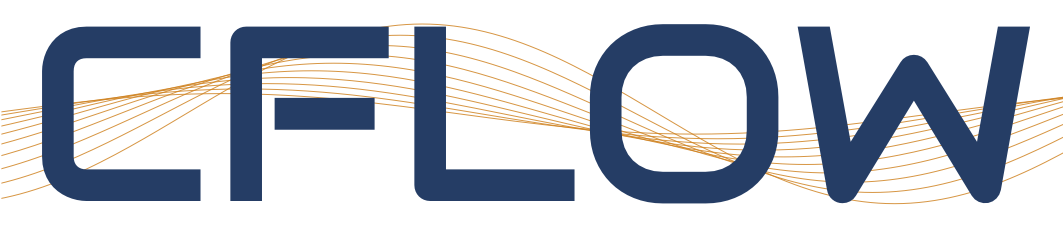In this exercise, you will investigate flow inside a convergent divergent nozzle using a quasi-1D approach and unsteady wave motion using the 1D solver available in CFLOW. In total three tasks should be solved of which the first deals with the flow in a convergent-divergent nozzle, the second problem is a shock tube and in the third task a traveling acoustic wave is investigated.

OBS Read chapters 5-7 in the course book before starting with the exercise!

When you have done the assignment, you should write a short report and hand in for assessment of this course element (instructions at the bottom of this page).

The CFLOW solver is a solver for simulation of compressible flows based on an explicit time-marching finite-volume-method (FVM) approach for which you can find a thorough description in the lectures on time-marching methods (Lectures 13 and 14). In order to be able to solve the tasks, you will have to make changes to the solver settings in accordance with the task description such as tube geometry, number of cells, number of time steps, boundary conditions, and initial flow properties. In order for the solver to run in a stable manner, you will also need to make changes to the solver numerics settings in some of the cases. In cases with steep gradients or discontinuities, extra damping will be needed. The extra damping is activated in the Numerics section. Pressure damping is used for shocks and density damping is for cases with steep temperature gradients.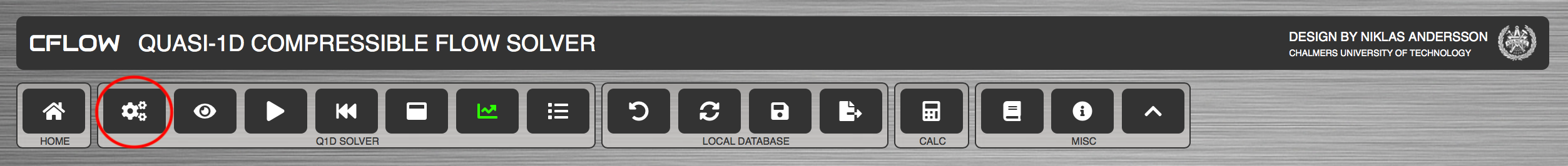Note that for compressible flows, the CFL-number is defined as:

$$CFL=\frac{\Delta t(c+|u|)}{\Delta x}$$

where $$\Delta t$$ is the time step size, $$c$$ is the speed of sound, $$u$$ is the fluid velocity, and $$\Delta x$$ is the local cell size. Since the solver uses explicit time marching, it is important that the CFL-number is below unity, otherwise the computation may diverge. You can find more details on the numerics of compressible flow solvers in the lecture notes on time-marching methods.

Detailed description of the solver settings and solver functionality can be found in the solver manual.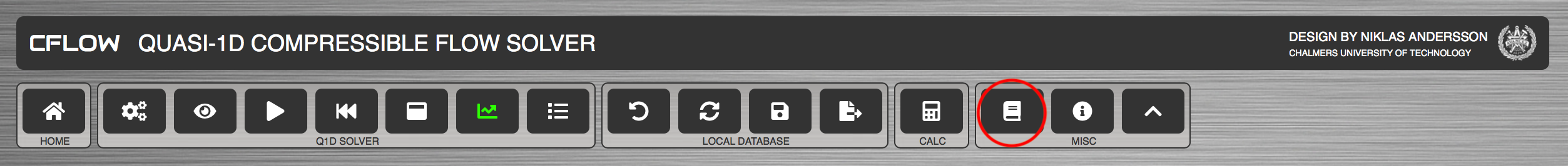##### Computational Domain

The figure below shows a schematic representation of the computational domain in CFLOW. Note that the solver is of finite-volume-method (FVM) type, which means that it solves for cell averages of flow properties.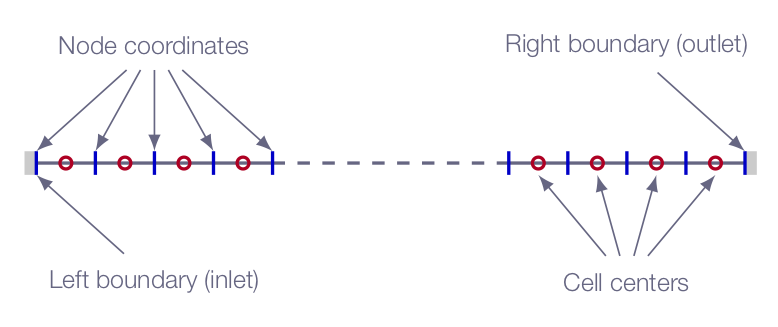##### Convergence

The convergence history is visualized in the solver log. Depending on task, the number of iterations needed in order to reach convergence will vary. In case the solver is not converged when the specified number of iterations are completed, increase the number of iterations and restart the solver.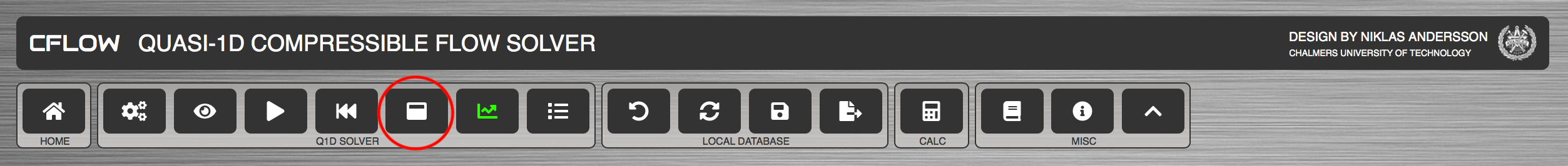##### Postprocessing

CFLOW will produce graphs of $$\rho$$, $$u$$, $$p$$, $$T$$, $$M$$, $$p_o$$, and $$T_o$$ for each obtained solution. Study the graphs and make sure that you can explain what you see.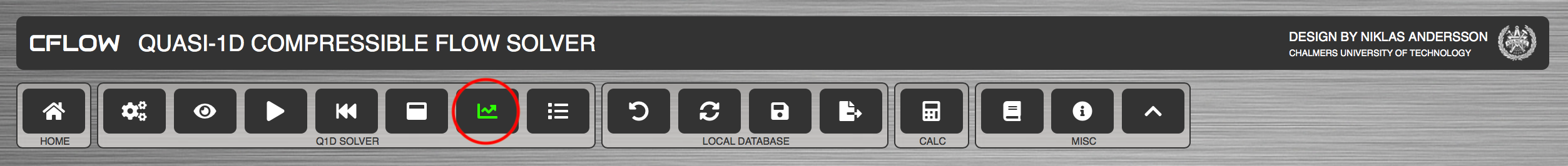##### Export

When done with one of the tasks below, save the CFLOW state to a JavaScript data base file (JSON) using the export functionality. The file can later be used to restore the solver state and should be submitted in Canvas along with the report.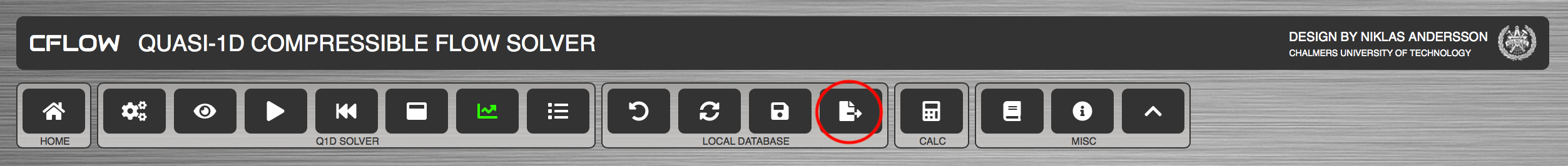All of the following tasks (taken from the course book) should be solved both analytically and numerically. Note that in order to be able to apply the correct boundary conditions in the numerical simulations, you will need to solve the problems analytically first!

### Task 1 - Quasi-1D simulation of the flow in a convergent-divergent nozzle

Problem 5.11

Consider a convergent-divergent duct with exit and throat areas of 0.5 m$$^2$$ and 0.25 m$$^2$$, respectively. The inlet reservoir pressure is 1.0 atm and the exit static pressure is 0.6 atm. For the numerical simulation, assume the reservoir temperature to be 288 K. Compare your simulation results with your calculations. The nozzle inlet area can be set to 0.3 m$$^2$$.

1. Setup the convergent-divergent nozzle problem as specified above in CFLOW. How do the results compare with your calculations? Compare e.g. $$A/A^\ast$$.
2. Calculate the pressure downstream of the nozzle exit which results in Mach=1 at the throat but subsonic in the entire divergent part of the nozzle. Run the case in the quasi-1D flow solver.
3. Calculate the pressure downstream of the nozzle exit below which the flow will be supersonic throughout the entire divergent part of the nozzle. Run the case in the quasi-1D flow solver.

Use appropriate boundary conditions for the three different cases.

Remember to export the solver state to a JSON-file when you are done with the task.

### Task 2 - shock tube

Problem 7.10

The driver and driven gases of a pressure-driven shock tube are both air at 300.0 K. The diaphragm pressure ratio $$p_4/p_1$$ is 5.0. In the CFLOW solver setup, use a constant area tube with 200 cells and solid walls on both boundaries, and make a piecewise constant initial solution (as you did for the normal shock problem in assignment 1). Furthermore, assume the driver section initial pressure to be $$p_4$$=500000 Pa.

Solve the problem analytically and compare with the results obtained with CFLOW.

You should calculate and compare

• The shock speed
• The head and tail speed of the expansion wave
• The velocity of the gas behind the shock
• The velocity of the reflected shock

One way of finding the shock, head, and tail velocities is produce several solutions with a few timesteps between (run the solver multiple times). By default, all solutions are saved and can be plotted in the same graphs for comparison purposes using the flow data archive functionality in CFLOW post. The speed can be estimated from the distance travelled from the center of the tube. For stability, use extra artificial numerical damping since there will be both strong shocks and contact discontinuities (pressure damping = 0.5, density damping=2).

Remember to export the solver state to a JSON-file when you are done with the task.

### Task 3 - traveling acoustic wave

Make a simulation of a harmonic acoustic wave in a constant area tube. Use periodic boundary condition and initialize a harmonic wave using the superimposed acoustic perturbation functionality in CFLOW (under Initial Flow Field in Settings).

• The tone should be a standard A440 (f=440 Hz) tone
• The length of the tube should be the same as the wave length ($$\lambda$$)
• The wave should travel a length corresponding to 10 wavelengths during the specified number of time steps

At approximately what sound pressure level does nonlinear effects become significant? Can this be estimated analytically, and if so how?

#### Formulas

The relation between the wave length $$\lambda$$ (m), the speed of sound in the undisturbed fluid $$a_{\infty}$$ (m/s), and the frequency $$f$$ (Hz) is as follows:

$$\lambda=\frac{a_{\infty}}{f}$$

The sound pressure level $$L_p$$ (dB) is calculated according to:

$$L_p=20\log_{10}\left(\frac{p_{rms}}{p_{ref}}\right)$$

where $$p_{rms}$$ is the root-mean-square of the pressure signal, and $$p_{ref}$$ is a reference pressure (here we used the value $$p_{ref}=2 \times 10^{-5}$$ Pa).

For harmonic waves the equation above can be rewritten as:

$$L_p=20\log_{10}\left(\frac{\Delta p}{p_{ref}\sqrt{2}}\right)$$

where $$\Delta p$$ is the amplitude of the pressure harmonic wave (Pa).

#### Hints

The time step $$\Delta t$$ is calculated as:

$$\Delta t=\frac{10}{f N}$$

where $$N$$ is the specified number of time steps. Set the number of time steps to $$N$$=1500.

Note!

$$N$$=1500 works if you use $$N_{cells}$$=100. If you, for example, set $$N_{cells}$$=200 and $$N$$=1500, the CFL number will be greater than 1.0, which will lead to solver instabilities.

$$\Delta t=\dfrac{10}{f N}$$ $$\Delta x=\dfrac{L}{N_{cells}}=\dfrac{\lambda}{N_{cells}}=\dfrac{a_\infty}{f N_{cells}}$$ $$CFL=\dfrac{\Delta t(|u|+a)}{\Delta x}=\dfrac{10N_{cells}(|u|+a)}{aN}\approx\left\{u\ll a,\ a=a_\infty\right\}\approx\dfrac{10N_{cells}}{N}$$

The table below gives CFL numbers for a range of values of $$N$$ and $$N_{cells}$$

$$N$$=1000 $$N$$=1250 $$N$$=1500 $$N$$=1750 $$N$$=2000 $$N$$=2250 $$N$$=2500
$$N_{cells}$$=100 1.00 0.80 0.67 0.57 0.50 0.44 0.40
$$N_{cells}$$=150 1.50 1.20 1.00 0.86 0.75 0.67 0.60
$$N_{cells}$$=200 2.00 1.60 1.33 1.14 1.00 0.88 0.80
$$N_{cells}$$=250 2.50 2.00 1.67 1.43 1.25 1.11 1.00

• To compare the profile of the sound wave for different sound pressure levels, mark the flow fields that you want to compare in the flow data archive found in CFLOW post
• For a better comparison use the normalize functionality which will normalize each profile using the maximum value

Remember to export the solver state to a JSON-file when you are done with the task.

### Assignment Report

In order to complete the assignment, a report should be written and handed in for assessment. Add a section in the report for each of the tasks above including:

1. Given data
2. Analytical solution
• Hand-written calculations can be included as images
3. CFLOW solver settings
1. Geometry settings
2. Mesh properties
3. Numerics
• numerical scheme
• artificial damping (if applicable)
• time integration
4. Run parameters
5. Gas properties
6. Initial flow field
7. Boundary conditions
• selected boundary condition type and specified values for both left and right boundary
8. Sources
4. Solver convergence
• Residual plot
• Do you consider the solution to be converged enough? Justify your answer
• Where there any problems getting the solver to converge for this specific case. If so, speculate on the cause for this behavior.
5. Results
• Flow field plots (Mach number, Temperature, Pressure)
• Additional flow field plots if it shows something of interest (for example total pressure and/or total temperature)
• Comment on what you see (specific flow features that can be observed)
• Do the results make sense?
6. Discussion
• Are there any significant deviations from the analytical/theoretical solution? If so, what is the reason for the deviations? (Might be difficult to answer exactly but a speculation will do)
• Are there any indications of numerical errors in the solution?
• wiggles in flow field data
• changes in total temperature for cases that should be adiabatic
• changes in total pressure for cases that should be isentropic
• other indications of numerical errors
If you see anything in your solutions that you think is an indication of numerical errors, try to explain why those errors appears.

When done, submit the report along with the exported JSON-files in Canvas